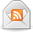# What is the Rule of 72?

Posted by Don on October 16, 2013

Have you heard of the Rule of 72? No it’s not something related to Breaking Bad. It’s a math formula. Before you go running from this page, hear me out. I’ll do my best to make this math lesson as easy and enjoyable as possible.

### What is the Rule of 72?

At its core, the rule of 72 is way to determine how long it will take for your money to double given an interest rate. Here is the formula:

Years to Double = 72 / Interest Rate

I told you this math was easy! Let’s take a look at a few examples so that we can see exactly how this formula works.

### Rule of 72 Examples

Example 1: Jane is earning 8% annually on her money. To find out how long it will take for her money to double, we take 72 and divide by 8. Note that if you are using a calculator for this you do not enter in 8% or .08. It is simply 8. So, 72 divided by 8 equals 9. This means it will take 9 years for Jane to double her money.

Example 2: Bob is earning 15% annually his money. To find out how long it will take for his money to double, we take 72 and divide by 15. If we take 72 divided by 15 (remember to just take 15 and not 15% or .15) it equals 4.8. This means it will take 4.8 years for Bob to double his money.

Example 3: Mike is earning 5% monthly his money. To find out how long it will take for his money to double, we take 72 and divide by 5. If we take 72 divided by 5 it equals 14.4. This means it will take 14.4 months for Mike to double his money. Note that it doesn’t matter if we are compounding monthly as in this example or annually in the examples above. The answer will give us years or months. In this case, our answer of 14.4 months can be divided by 12 to get years. In years, 14.4 months is 1.2 years.

Example 4: Joe enjoys economics and sees that inflation is currently 3%. At this rate, he wants to know how long it will take until his beloved potato chips will double in price. If Joe take 72 divided by 3, he will see that the price of a bag of his favorite potato chips will be double their current price in 24 years.

### Why Use The Rule of 72?

You may be asking why even use the rule of 72. This is a great question. With compound interest you earn interest on your original principal as well as the interest you earn. The formula for this can get cumbersome when you take it out a few years. If you have a calculator, the math isn’t too difficult. But where you really run into trouble is trying to figure out how long it will take for your money to double.

I’ll spare you the math, but trying to figure out the time it will take for your money to double on a calculator requires the use of the “log” key on your calculator. As a result, it is virtually impossible to do the math in your head. With the rule of 72, it makes determining how long it will take for your money to double much easier.

### One More Feature of the Rule of 72

As with any math equation, we can turn it around to figure out what interest rate we need to earn on our money, given a period of time. Let’s say you have \$100,000 and you want your money to double to \$200,000 in four years. What interest rate do you need to meet your goal?

To find the answer, we take 72 and divide by 4. The answer is 18. This means you need to earn 18% on your money if you want it to double in 4 years.

### More Rules to Play With

Some of you reading this might not be content with having your money double. You want it to triple or quadruple in amount. Well, I have great news for you!

Rule of 115. If you want to see how long it will take your money to triple in amount, you can use the rule of 115.

Rule of 144. For those interested in quadrupling your money, then the rule of 144 is your go to formula. Both work the exact same way as the rule of 72.

### Final Thoughts

I hope you found this math lesson easy and entertaining. If not, hey, it’s math. There’s not too much you can do to make it fun.

While this calculation is quick and easy, it may not prove to be exact for you. This isn’t to say the formula isn’t reliable, because it is. It’s just that the formula doesn’t take into account taxes and other factors that could cause your real interest rate to be lower. But, it will give you a fairly accurate assessment of how long it will take your money to double.

### More Financial Calculations